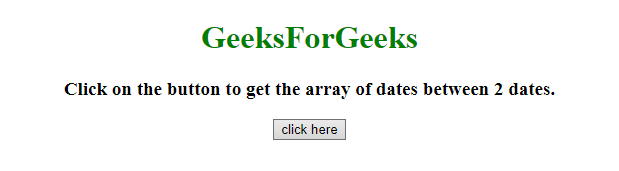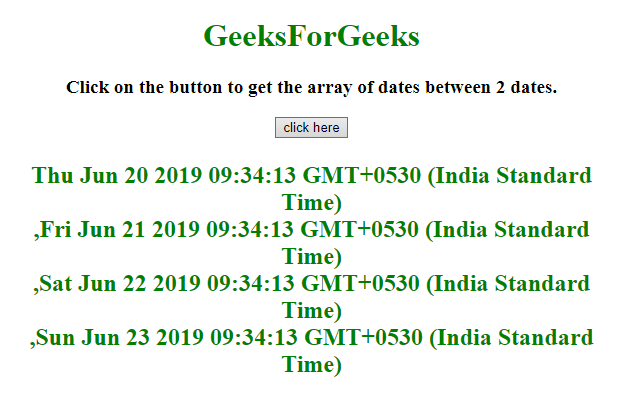# How to store all dates in an array present in between given two dates in JavaScript ?

Given two dates and the task is to get the array of dates between the two given dates using JavaScript.

Approach 1:

• Select the first and last date and store into variable.
• Check if start date is less then stop date then push the currentdate in an array and increment its value by 1 day.
• Repeat above step until currentDate equal to the last date.

Example: In this example, the array of dates is determined by the above approach.

 `  ` `<``html``>  ` ` `  `<``head``>  ` `    ``<``title``>  ` `        ``How to get the array of dates between ` `        ``two dates in JavaScript ? ` `    `` ` `  ` ` `  `<``body` `style` `= ``"text-align:center;"``>  ` `     `  `    ``<``h1` `style` `= ``"color:green;"` `>  ` `        ``GeeksForGeeks ` `    `` ` `     `  `    ``<``p` `id` `= ``"GFG_UP"` `style` `= ` `        ``"font-size: 19px; font-weight: bold;"``> ` `    `` ` `     `  `    ``<``button` `onclick` `= ``"GFG_Fun()"``> ` `        ``click here ` `    `` ` `     `  `    ``<``p` `id` `= ``"GFG_DOWN"` `style` `= ` `        ``"color: green; font-size: 24px; font-weight: bold;"``> ` `    `` ` `     `  `    ``<``script``> ` `        ``var el_up = document.getElementById("GFG_UP"); ` `        ``var el_down = document.getElementById("GFG_DOWN"); ` `     `  `        ``el_up.innerHTML = "Click on the button to get the " ` `                    ``+ "array of dates between 2 dates."; ` `         `  `        ``Date.prototype.addDay = function(days) { ` `            ``var date = new Date(this.valueOf()); ` `            ``date.setDate(date.getDate() + days); ` `            ``return date; ` `        ``} ` `         `  `        ``function getDate(strDate, stpDate) { ` `            ``var dArray = new Array(); ` `            ``var cDate = strDate; ` `            ``while (cDate <= stpDate) { ` `                 `  `                ``// Adding the date to array ` `                ``dArray.push(new Date (cDate) + '<``br``>');  ` `                 `  `                ``// Increment the date by 1 day ` `                ``cDate = cDate.addDay(1);  ` `            ``} ` `            ``return dArray; ` `        ``} ` `         `  `        ``function GFG_Fun() { ` `            ``var startDate = new Date(); ` `             `  `            ``// Making lastDate equal to 4 more days ` `            ``// from startDate. ` `            ``var endDate = startDate.addDay(4);  ` `            ``el_down.innerHTML = getDate(startDate, endDate); ` `        ``} ` `    ``  ` `  ` ` `  `     `

Output:

• Before clicking on the button:• After clicking on the button:Approach 2:

• Get the first and last date and store it into variable.
• Calculate 1 day equivalent in milliseconds called _1Day.
• Set a variable equal to start date, called ms
• Push ms (milli-seconds) in form of date in an array and increment its value by _1Day.
• Repeat above step until ms equal to the last date.

Example: In this example, the array of dates is determined by the above approach.

 `  ` `<``html``>  ` ` `  `<``head``>  ` `    ``<``title``>  ` `        ``How to get the array of dates between ` `        ``two dates in JavaScript ? ` `    `` ` `  ` ` `  `<``body` `style` `= ``"text-align:center;"``>  ` ` `  `    ``<``h1` `style` `= ``"color:green;"` `>  ` `        ``GeeksForGeeks ` `    `` ` ` `  `    ``<``p` `id` `= ``"GFG_UP"` `style` `= ` `        ``"font-size: 19px; font-weight: bold;"``> ` `    `` ` `     `  `    ``<``button` `onclick` `= ``"GFG_Fun()"``> ` `        ``click here ` `    `` ` `     `  `    ``<``p` `id` `= ``"GFG_DOWN"` `style` `=  ` `        ``"color: green; font-size: 24px; font-weight: bold;"``> ` `    `` ` `     `  `    ``<``script``> ` `        ``var el_up = document.getElementById("GFG_UP"); ` `        ``var el_down = document.getElementById("GFG_DOWN"); ` `         `  `        ``el_up.innerHTML = "Click on the button to get the " ` `                ``+ "array of dates between 2 dates."; ` `         `  `        ``Date.prototype.addDay = function(days) { ` `            ``var date = new Date(this.valueOf()); ` `            ``date.setDate(date.getDate() + days); ` `            ``return date; ` `        ``} ` `         `  `        ``function getDates( date1, date2 ) { ` `            ``var _1Day = 24*3600*1000; ` `             `  `            ``// Date[] keeps all the dates ` `            ``for (var date = [], ms = date1 * 1, last = date2 * 1; ` `                            ``ms < ``last` `; ms += _1Day) {  ` `                                 `  `                ``// Adding ms to the date and ms+= _1Day ` `                ``// increments the date by 1 day ` `                ``date.push( new Date(ms) + '
'); ` `            ``} ` `             `  `            ``return date; ` `        ``} ` `         `  `        ``function GFG_Fun() { ` `            ``var startDate = new Date(); ` `             `  `            ``// Making lastDate equal to 4 more days ` `            ``// from startDate ` `            ``var endDate = startDate.addDay(4);  ` `            ``el_down.innerHTML = getDates(startDate, endDate); ` `        ``} ` `    ``  ` `  ` ` `  ` `

Output:

• Before clicking on the button:• After clicking on the button:My Personal Notes arrow_drop_upCheck out this Author's contributed articles.

If you like GeeksforGeeks and would like to contribute, you can also write an article using contribute.geeksforgeeks.org or mail your article to contribute@geeksforgeeks.org. See your article appearing on the GeeksforGeeks main page and help other Geeks.

Please Improve this article if you find anything incorrect by clicking on the "Improve Article" button below.